SNOSAY9G September   2008  – February 2016

PRODUCTION DATA.

1. Features
2. Applications
3. Description
4. Revision History
5. Pin Configuration and Functions SC-70 and VSSOP references from LMP2021 pinout descriptions
6. Specifications
7. Detailed Description
8. Application and Implementation
1. 8.1 Application Information
2. 8.2 Typical Application
9. Power Supply Recommendations
10. 10Layout
11. 11Device and Documentation Support
12. 12Mechanical, Packaging, and Orderable Information

• D|8
• DBV|5

## 6 Specifications

### 6.1 Absolute Maximum Ratings(1)(2)

MIN MAX UNIT
VIN Differential –VS VS
Supply Voltage (VS = V+ – V) 6.0 V
All Other Pins V+ + 0.3 V − 0.3 V
Output Short-Circuit Duration to V+ or V(3) 5 seconds
Junction Temperature(4) 150 °C
Soldering Information    Infrared or Convection (20 sec) 235 °C
Wave Soldering Lead Temperature (10 sec) 260 °C
Tstg Storage temperature range −65 150 °C
(1) Absolute Maximum Ratings indicate limits beyond which damage to the device may occur. Recommended Operating Conditions indicate conditions for which the device is intended to be functional, but specific performance is not ensured. For ensured specifications and the test conditions, see the Electrical Characteristics Tables.
(2) If Military/Aerospace specified devices are required, please contact the TI Sales Office/ Distributors for availability and specifications.
(3) Package power dissipation should be observed.
(4) The maximum power dissipation is a function of TJ(MAX), θJA, and TA. The maximum allowable power dissipation at any ambient temperature is PD = (TJ(MAX) - TA)/ θJA. All numbers apply for packages soldered directly onto a PC board.

### 6.2 ESD Ratings

VALUE UNIT
V(ESD) Electrostatic discharge Human body model (HBM), per ANSI/ESDA/JEDEC JS-001, all pins(1) ±2000 V
Charged device model (CDM), per JEDEC specification JESD22-C101, all pins(2) ±1000
Machine model ±200
(1) JEDEC document JEP155 states that 500-V HBM allows safe manufacturing with a standard ESD control process.
(2) JEDEC document JEP157 states that 250-V CDM allows safe manufacturing with a standard ESD control process.

### 6.3 Recommended Operating Conditions

over operating free-air temperature range (unless otherwise noted)
MIN MAX UNIT
Temperature Range −40 125 °C
Supply Voltage (VS = V+ – V) 2.2 5.5 V

### 6.4 Thermal Information

THERMAL METRIC(1) LMP2021, LMP2022 LMP2021 LMP2022 UNIT
D DBV DGK
8 PINS 5 PINS 8 PINS
RθJA Junction-to-ambient thermal resistance 106 164 217 °C/W

### 6.5 Electrical Characteristics: 2.5 V(1)

Unless otherwise specified, all limits are ensured for TA = 25°C, V+ = 2.5 V, V = 0 V, VCM = V+/2, RL >10 kΩ to V+/2.
PARAMETER TEST CONDITIONS MIN(3) TYP(2) MAX(3) UNIT
VOS Input Offset Voltage –5 –0.9 5 μV
–40°C ≤ TJ ≤ 125°C –10 10
TCVOS Input Offset Voltage Drift(4) –0.02 0.001 0.02 μV/°C
IB Input Bias Current –100 ±23 100 pA
–40°C ≤ TJ ≤ 125°C –300 300
IOS Input Offset Current –200 ±57 200 pA
–40°C ≤ TJ ≤ 125°C –250 250
CMRR Common Mode Rejection Ratio −0.2 V ≤ VCM ≤ 1.7 V, 0 V ≤ VCM ≤ 1.5 V 105 141 dB
−0.2 V ≤ VCM ≤ 1.7 V, 0 V ≤ VCM ≤ 1.5 V, –40°C ≤ TJ ≤ 125°C 102
CMVR Input Common-Mode Voltage Range Large Signal CMRR ≥ 105 dB −0.2 1.7 V
Large Signal CMRR ≥ 102 dB, –40°C ≤ TJ ≤ 125°C 0 1.5
EMIRR Electro-Magnetic Interference Rejection Ratio(3) IN+ and IN− VRF-PEAK = 100 mVP (−20 dBVP)
f = 400 MHz
40 dB
VRF-PEAK = 100 mVP (−20 dBVP)
f = 900 MHz
48
VRF-PEAK = 100 mVP (−20 dBVP)
f = 1800 MHz
67
VRF-PEAK = 100 mVP (−20 dBVP)
f = 2400 MHz
79
PSRR Power Supply Rejection Ratio 2.5 V ≤ V+ ≤ 5.5 V, VCM = 0 115 130 dB
2.5 V ≤ V+ ≤ 5.5 V, VCM = 0 , –40°C ≤ TJ ≤ 125°C 112
2.2 V ≤ V+ ≤ 5.5 V, VCM = 0 110 130
AVOL Large Signal Voltage Gain RL = 10 kΩ to V+/2, VOUT = 0.5 V to 2 V 124 150 dB
RL = 10 kΩ to V+/2, VOUT = 0.5 V to 2 V, –40°C ≤ TJ ≤ 125°C 119
RL = 2 kΩ to V+/2, VOUT = 0.5 V to 2 V 120 150
RL = 2 kΩ to V+/2, VOUT = 0.5 V to 2 V, –40°C ≤ TJ ≤ 125°C 115
VOUT Output Swing High RL = 10 kΩ to V+/2 38 50 mV
from either rail
RL = 10 kΩ to V+/2, –40°C ≤ TJ ≤ 125°C 70
RL = 2 kΩ to V+/2 62 85
RL = 2 kΩ to V+/2, –40°C ≤ TJ ≤ 125°C 115
Output Swing Low RL = 10 kΩ to V+/2 30 45
RL = 10 kΩ to V+/2, –40°C ≤ TJ ≤ 125°C 55
RL = 2 kΩ to V+/2 58 75
RL = 2 kΩ to V+/2, –40°C ≤ TJ ≤ 125°C 95
IOUT Linear Output Current
Sourcing, VOUT = 2 V 30 50 mA
Sinking, VOUT = 0.5 V 30 50
IS Supply Current Per Amplifier 0.95 1.10 mA
Per Amplifier, –40°C ≤ TJ ≤ 125°C 1.37
SR Slew Rate(5) AV = +1, CL = 20 pF, RL = 10 kΩ
VO = 2 VPP
2.5 V/μs
GBW Gain Bandwidth Product CL = 20 pF, RL = 10 kΩ 5 MHz
GM Gain Margin CL = 20 pF, RL = 10 kΩ 10 dB
ΦM Phase Margin CL = 20 pF, RL = 10 kΩ 60 deg
CIN Input Capacitance Common Mode 12 pF
Differential Mode 12
en Input-Referred Voltage Noise Density f = 0.1 kHz or 10 kHz, AV = 1000 11 nV/√Hz
f = 0.1 kHz or 10 kHz, AV = 100 15
Input-Referred Voltage Noise 0.1 Hz to 10 Hz 260 nVPP
0.01 Hz to 10 Hz 330
In Input-Referred Current Noise f = 1 kHz 350 fA/√Hz
tr Recovery time to 0.1%, RL = 10 kΩ, AV = −50,
VOUT = 1.25 VPP Step, Duration = 50 μs
50 µs
CT Cross Talk LMP2022, f = 1 kHz 150 dB
(1) Electrical Table values apply only for factory testing conditions at the temperature indicated. Factory testing conditions result in very limited self-heating of the device such that TJ = TA. No specification of parametric performance is indicated in the electrical tables under conditions of internal self-heating where TJ > TA.
(2) Typical values represent the most likely parametric norm at the time of characterization. Actual typical values may vary over time and will also depend on the application and configuration. The typical values are not tested and are not ensured on shipped production material.
(3) All limits are specified by testing, statistical analysis or design.
(4) Offset voltage temperature drift is determined by dividing the change in VOS at the temperature extremes by the total temperature change.
(5) The number specified is the average of rising and falling slew rates and is measured at 90% to 10%.

### 6.6 Electrical Characteristics: 5 V(1)

Unless otherwise specified, all limits are ensured for TA = 25°C, V+ = 5 V, V = 0 V, VCM = V+/2, RL > 10 kΩ to V+/2.
PARAMETER TEST CONDITIONS MIN(3) TYP(2) MAX(3) UNIT
VOS Input Offset Voltage –5 −0.4 5 μV
–40°C ≤ TJ ≤ 125°C –10 10
TCVOS Input Offset Voltage Drift(2) –0.02 −0.004 0.02 μV/°C
IB Input Bias Current –100 ±25 100 pA
–40°C ≤ TJ ≤ 125°C –300 300
IOS Input Offset Current –200 ±48 200 pA
–40°C ≤ TJ ≤ 125°C –250 250
CMRR Common Mode Rejection Ratio −0.2 V ≤ VCM ≤ 4.2 V, 0 V ≤ VCM ≤ 4.0 V 120 139 dB
−0.2 V ≤ VCM ≤ 4.2 V, 0 V ≤ VCM ≤ 4.0 V, –40°C ≤ TJ ≤ 125°C 115
CMVR Input Common-Mode Voltage Range Large Signal CMRR ≥ 120 dB –0.2 4.2 V
Large Signal CMRR ≥ 115 dB, –40°C ≤ TJ ≤ 125°C 0 4.0
EMIRR Electro-Magnetic Interference Rejection Ratio(3) IN+ and IN− VRF-PEAK = 100 mVP (−20 dBVP)
f = 400 MHz
58 dB
VRF-PEAK = 100 mVP (−20 dBVP)
f = 900 MHz
64
VRF-PEAK = 100 mVP (−20 dBVP)
f = 1800 MHz
72
VRF-PEAK = 100 mVP (−20 dBVP)
f = 2400 MHz
82
PSRR Power Supply Rejection Ratio 2.5 V ≤ V+ ≤ 5.5 V, VCM = 0 115 130 dB
2.5 V ≤ V+ ≤ 5.5 V, VCM = 0, –40°C ≤ TJ ≤ 125°C 112
2.2 V ≤ V+ ≤ 5.5 V, VCM = 0 110 130
AVOL Large Signal Voltage Gain RL = 10 kΩ to V+/2, VOUT = 0.5 V to 4.5 V 125 160 dB
RL = 10 kΩ to V+/2, VOUT = 0.5 V to 4.5 V, –40°C ≤ TJ ≤ 125°C 120
RL = 2 kΩ to V+/2, VOUT = 0.5 V to 4.5 V 123 160
RL = 2 kΩ to V+/2, VOUT = 0.5 V to 4.5 V, –40°C ≤ TJ ≤ 125°C 118
VOUT Output Swing High RL = 10 kΩ to V+/2 83 135 mV
from either rail
RL = 10 kΩ to V+/2, –40°C ≤ TJ ≤ 125°C 170
RL = 2 kΩ to V+/2 120 160
RL = 2 kΩ to V+/2, –40°C ≤ TJ ≤ 125°C 204
Output Swing Low RL = 10 kΩ to V+/2 65 80
RL = 10 kΩ to V+/2, –40°C ≤ TJ ≤ 125°C 105
RL = 2 kΩ to V+/2 103 125
RL = 2 kΩ to V+/2, –40°C ≤ TJ ≤ 125°C 158
IOUT Linear Output Current
Sourcing, VOUT = 4.5 V 30 50 mA
Sinking, VOUT = 0.5 V 30 50
IS Supply Current Per Amplifier 1.1 1.25 mA
Per Amplifier, –40°C ≤ TJ ≤ 125°C 1.57
SR Slew Rate(4) AV = +1, CL = 20 pF, RL = 10 kΩ
VO = 2 VPP
2.6 V/μs
GBW Gain Bandwidth Product CL = 20 pF, RL = 10 kΩ 5 MHz
GM Gain Margin CL = 20 pF, RL = 10 kΩ 10 dB
ΦM Phase Margin CL = 20 pF, RL = 10 kΩ 60 deg
CIN Input Capacitance Common Mode 12 pF
Differential Mode 12
en Input-Referred Voltage Noise Density f = 0.1 kHz or 10 kHz, AV= 1000 11 nV/√Hz
f = 0.1 kHz or 10 kHz, AV= 100 15
Input-Referred Voltage Noise 0.1 Hz to 10 Hz Noise 260 nVPP
0.01 Hz to 10 Hz Noise 330
In Input-Referred Current Noise f = 1 kHz 350 fA/√Hz
tr Input Overload Recovery time to 0.1%, RL = 10 kΩ, AV = −50,
VOUT = 2.5 VPP Step, Duration = 50 μs
50 μs
CT Cross Talk LMP2022, f = 1 kHz 150 dB
(1) Electrical Table values apply only for factory testing conditions at the temperature indicated. Factory testing conditions result in very limited self-heating of the device such that TJ = TA. No specification of parametric performance is indicated in the electrical tables under conditions of internal self-heating where TJ > TA.
(2) Offset voltage temperature drift is determined by dividing the change in VOS at the temperature extremes by the total temperature change.
(3) The EMI Rejection Ratio is defined as EMIRR = 20Log ( VRF-PEAK/ΔVOS).
(4) The number specified is the average of rising and falling slew rates and is measured at 90% to 10%.

### 6.7 Typical Characteristics

Unless otherwise noted: TA = 25°C, RL > 10 kΩ, VS= V+ – V, VS= 5 V, VCM = VS/2.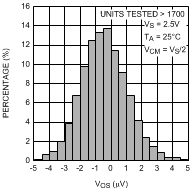Figure 1. Offset Voltage Distribution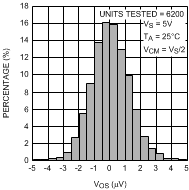Figure 3. Offset Voltage Distribution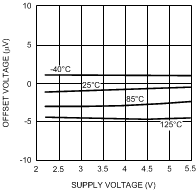Figure 5. Offset Voltage vs. Supply Voltage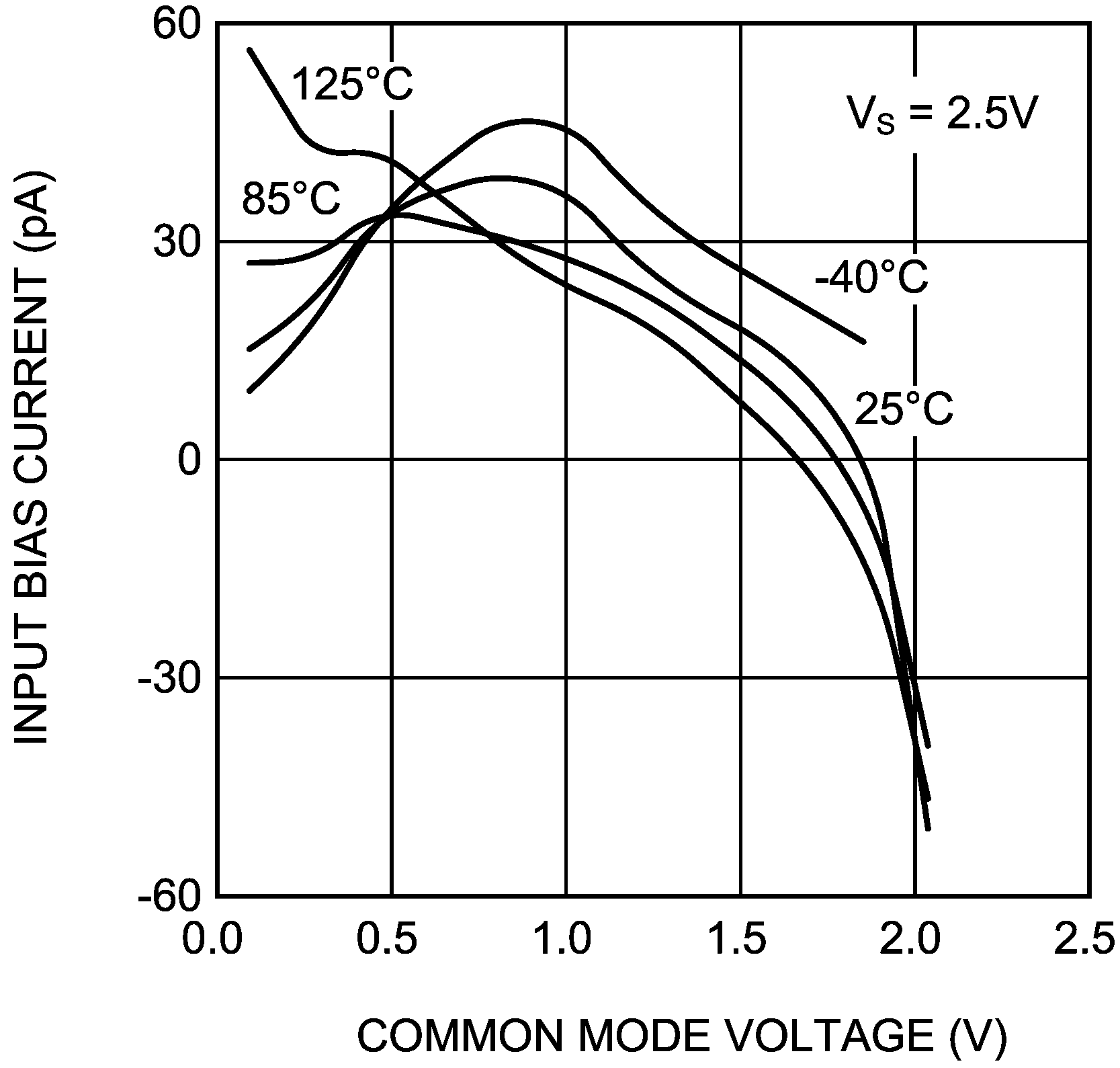Figure 7. Input Bias Current vs. VCM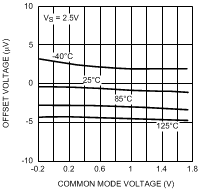Figure 9. Offset Voltage vs. VCM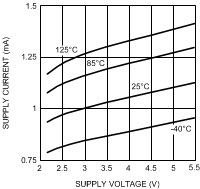Figure 11. Supply Current vs. Supply Voltage (Per Amplifier)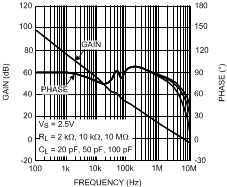Figure 13. Open Loop Frequency Response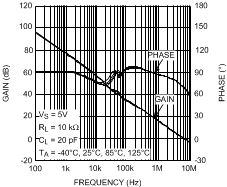Figure 15. Open Loop Frequency Response Over Temperature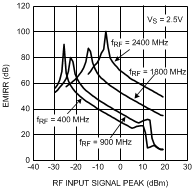Figure 17. EMIRR vs. Input Power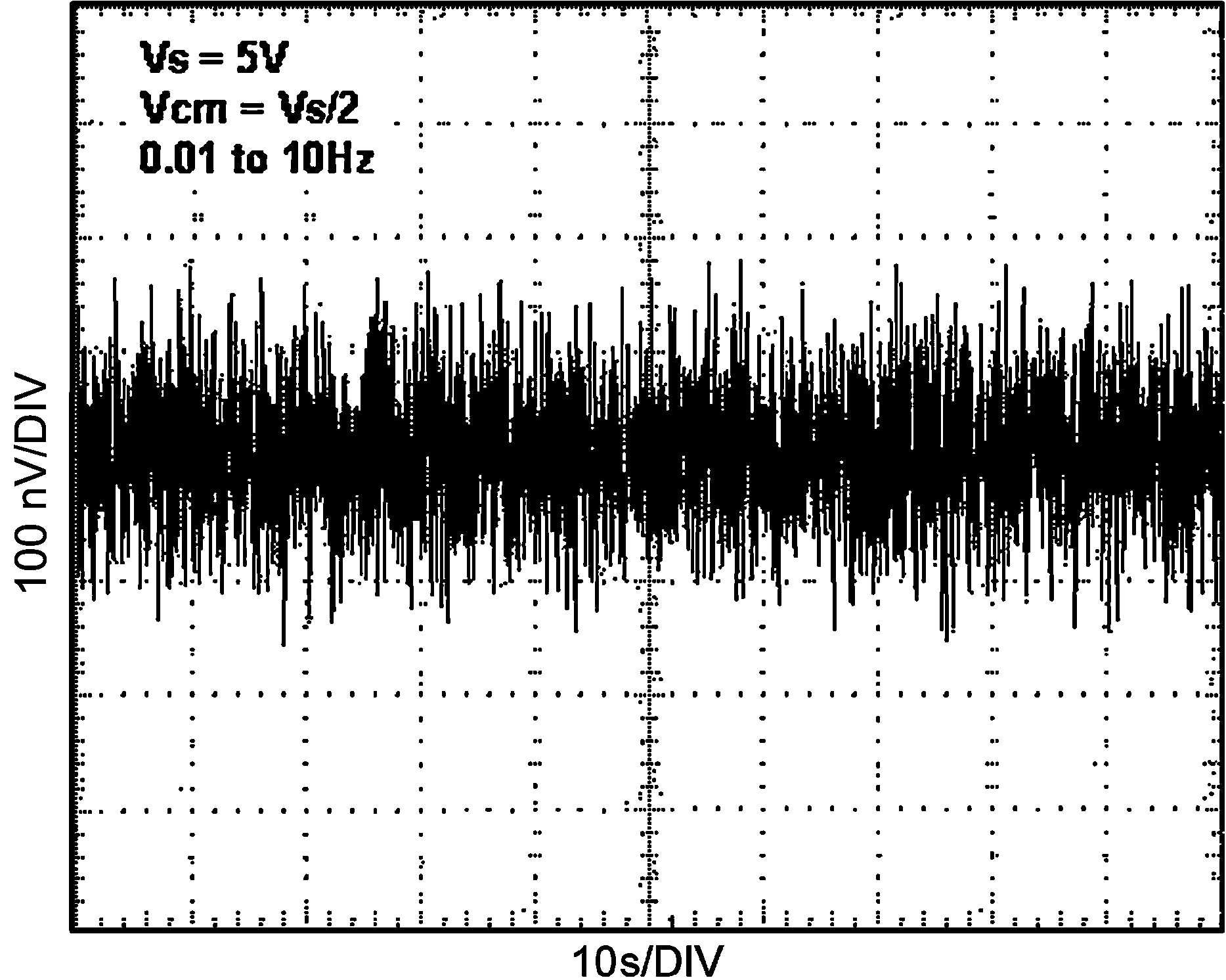Figure 19. Time Domain Input Voltage Noise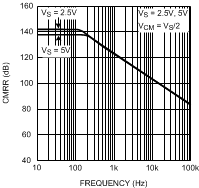Figure 21. CMRR vs. FrequencyFigure 23. Output Swing High vs. Supply Voltage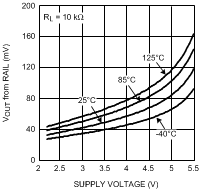Figure 25. Output Swing High vs. Supply Voltage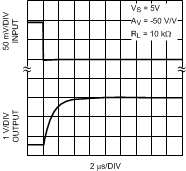Figure 27. Overload Recovery Time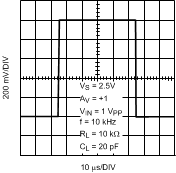Figure 29. Large Signal Step Response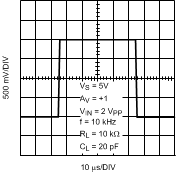Figure 31. Large Signal Step Response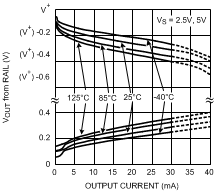Figure 33. Output Voltage vs. Output Current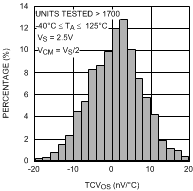Figure 2. TCVOS Distribution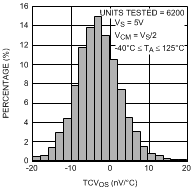Figure 4. TCVOS Distribution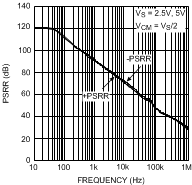Figure 6. PSRR vs. FrequencyFigure 8. Input Bias Current vs. VCM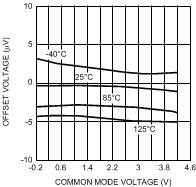Figure 10. Offset Voltage vs. VCM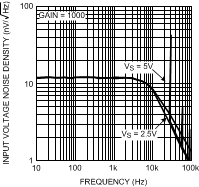Figure 12. Input Voltage Noise vs. Frequency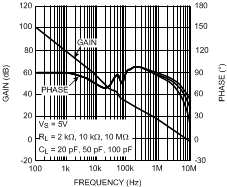Figure 14. Open Loop Frequency Response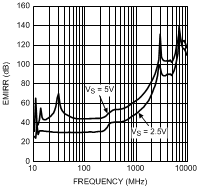Figure 16. EMIRR vs. Frequency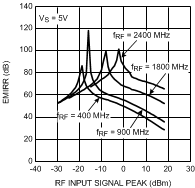Figure 18. EMIRR vs. Input Power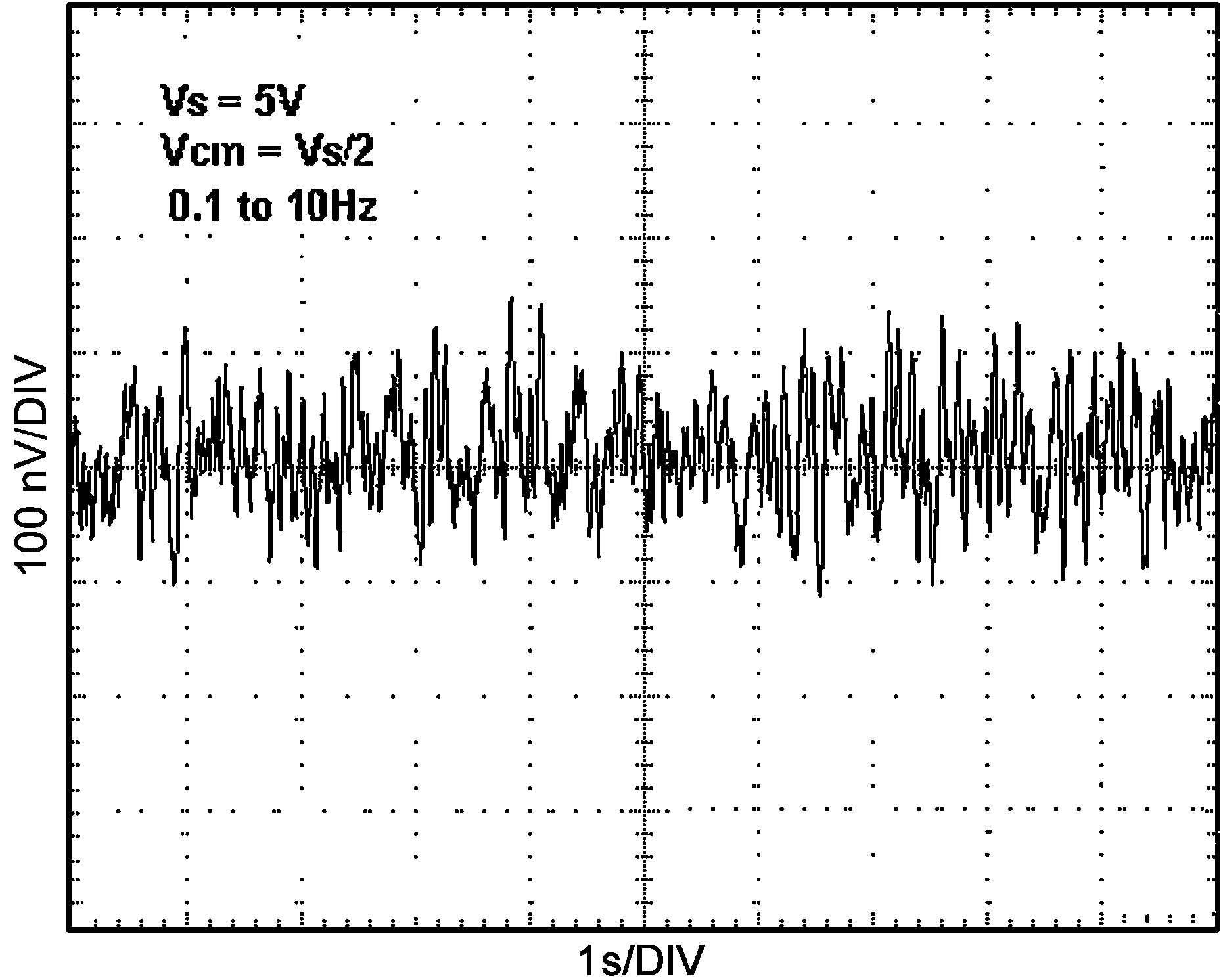Figure 20. Time Domain Input Voltage Noise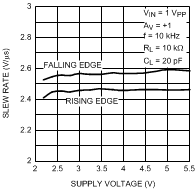Figure 22. Slew Rate vs. Supply VoltageFigure 24. Output Swing Low vs. Supply Voltage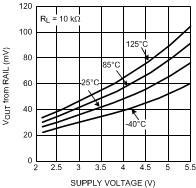Figure 26. Output Swing Low vs. Supply Voltage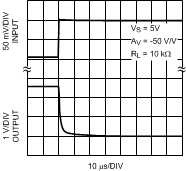Figure 28. Overload Recovery Time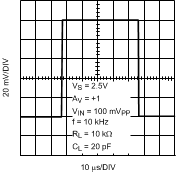Figure 30. Small Signal Step Response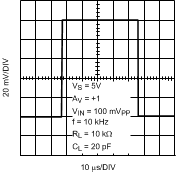Figure 32. Small Signal Step Response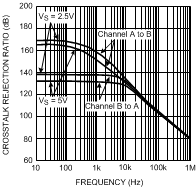Figure 34. Cross Talk Rejection Ratio vs. Frequency (LMP2022)